Graphing Derivatives The TI-83+/84+ calculators will produce graphs of the derivatives of functions. Graph the derivative of the function: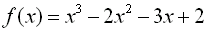Step 1: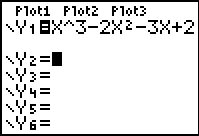Enter the function into Y1=. Arrow down so the cursor is in the Y2= entry space. Step 2: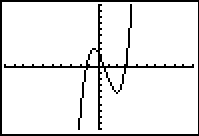GRAPH the original function to see that your viewing window gives a good representation of the graph. Step 3: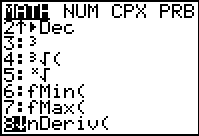We will be using the nDeriv command found under the MATH key.   Hit the MATH key and arrow down to choice #8 nDeriv(. Step 4: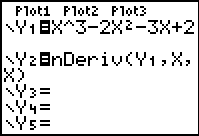The nDeriv command will appear in the Y2= entry space, where you left the cursor. We will be using the parameters nDeriv(Y1, X, X). Step 5: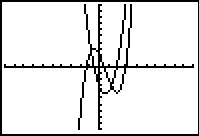Hit GRAPH. Step 6:To more clearly distinguish the derivative graph, set the graph style to a thicker line (or graph the derivative separately).